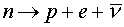Strong, weak and Electromagnetic forces

Nucleons interact through three different means. These are refered to a "forces" even though "interactions" might be a more appropriate term.

1. Strong force: This is a short-range attractive force. "Short range" refers to the fact that the force dies off exponentially in distance. This means that a nucleon is only affected by the strong force of its nearest neighbors. However, within that distance of less than 2.0 E-15 m, it overwhelms the other forces.

2. Weak force: The weak force is much weaker and much shorter range than the strong force. It is the magic wand that can change neutrons into protons, a process known as beta decay:. In this decay, a neutron decays to a proton, an electron, and an anti-neutrino. Beta- is an old-fashioned name for the electron e-. Another example of beta- decay is the decay of carbon 14 to nitrogen 14 + e- + anti-neutrino. There are related beta+ decays in which a proton changes into a neutron, an e+, and a neutrino. The e+ is the anti-particle of the electron: its old-fashioned name is beta+; its modern name is positron.

Nuclei prefer to have roughly equal numbers of neutrons and protons on account of the Pauli Exclusion Principle: nuclei that have an unbalanced ratio of neutrons to protons will radioactively decay into nuclei that are more balanced and thus have lower energies, by beta- or beta+ decay.

3. Electromagnetic force: The Coulomb force, which acts between protons, is a long-range force. For nucleons that are near one another in the nucleus, the coulomb interaction is dwarfed by the strong force. But in large nuclei, two protons at opposite ends of a nucleus continue to feel the repulsive effects of the coulomb interaction. The coulomb repulsion is responsible for the fact that heavy nuclei have more neutrons than protons.

Coulomb repulsion is also responsible for fission of large nuclei. If it were not for the electromagnetic force, nuclei could grow arbitrarily large.

Nuclear physics index      examples        Lecture index